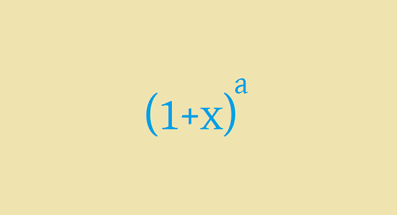# Continued Factorial Fractions

Calculus Level 1$1 + \frac{1}{1!} + \frac{1}{2!} + \frac{1}{3!} + \frac{1}{4!} + \ldots$ What is the value of this continued fraction correct up to 2 decimal places?

This problem is a part of set nested radicals

×